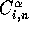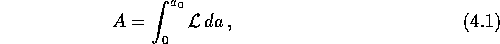Next: Integration Up: Numerical Methods Previous: Numerical Methods

# Introduction

Equation (3.35) should be understood as a multidimensional system of non-linear equations that puts constraints on the values of the coefficients.The coefficients may be determined by solving these equations using a root finding procedure, but, numerically, there are no good, general methods for solving systems of more than one non-linear equation. The reason is that we have to solve all the equations simultaneously; that is, finding the points where they all are zero. This is not a feasible task, especially since there is no information on the common curvature of the set of equations. To attain such information, we need the partial derivatives of equation (3.35), which is a rather computationally costly procedure.There are, however, efficient general techniques for finding stationary values of a function of many variables, a process which are often referred to as optimization. As seen in Chapter 3, locating stationary values are equivalent to finding a zero of the gradient vector in equation (3.28), which, at first glance, might not seem so different from zeroing a multidimensional function like the equations of motion in (3.35). But, there is one distinct difference: the gradient vector contain advantageous information on the direction of the stationary value, information that (3.35) does not have. The partial derivative of the action will aid the search for stationary values considerably, giving a more efficient algorithm than root finding. Therefore, in the quest of values for the coefficients which gives the correct motion of galaxies, it seems preferable to return to the Hamilton's principle:where the Lagrangianis given by equation (3.20) and time is given as the expansion parameter.

Optimization, or rather, in this case, unconstrained optimization, has developed rapidly since the advent of electronic computers in 1945, and a number of methods has been proposed over the years. For quadratic functions most of these methods do the job very well, even for large numbers of unknown variables. It is when we are dealing with non-quadratic functions that it starts to get more difficult. For a quadratic function, some of the optimization methods will be able to locate the extremum after only n iterations, n being the number of unknown variables. For non-quadratic functions, the number of iterations increase considerably, and it is not even certain that some of the methods will be able to find the extremum after an infinite number of iterations. The choice of method can therefore prove to be crucial, both for effectiveness, and for actually locating the extremum.

Optimization is made even more complex when dealing with non-linear functions, where there will be a number of stationary points: global, local and/or saddle points. The problem here is to distinguish between them, especially locating the global extrema.

Equation (4.1) has both these complicating factors, making optimization a central problem in the AVP. I will therefore take a closer look at some of the optimization methods in this chapter, trying to locate the ones that are applicable here. But, before doing that, we also need an effective numerical method for integrating equation (4.1) and its derivatives. The choice can also be of some importance in the attempt to reduce the computing time of the stationary values.Next: Integration Up: Numerical Methods Previous: Numerical Methods

Trond Hjorteland
Mon Jul 5 02:59:28 MET DST 1999